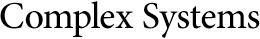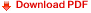## Computational Irreducibility and Computational AnalogyHervé Zwirn

UFR de Physique (LIED, Université Paris 7)
CMLA (ENS Cachan, France)
and
IHPST (CNRS, France)
herve.zwirn@gmail.com

#### Abstract

In a previous paper , we provided a formal definition for the concept of computational irreducibility (CIR), that is, the fact that for a function f from N to N it is impossible to compute f(n) without following approximately the same path as computing successively all the values f(i) from i=1 to n. Our definition is based on the concept of enumerating Turing machines (E-Turing machines) and on the concept of approximation of E-Turing machines, for which we also gave a formal definition. Here, we make these definitions more precise through some modifications intended to improve the robustness of the concept. We then introduce a new concept: the computational analogy, and prove some properties of the functions used. Computational analogy is an equivalence relation that allows partitioning the set of computable functions in classes whose members have the same properties regarding their CIR and their computational complexity.

https://doi.org/10.25088/ComplexSystems.24.2.149